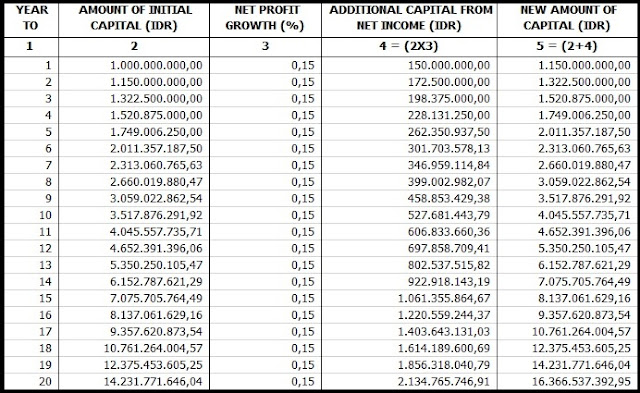# Double The Value Of Assets

DOUBLE THE VALUE OF ASSETS

Investment basically aims to multiply the value of the assets that you currently have to be many times in the future. This can be realized if there is a growth in the value of a number of assets. Asset value growth will occur if the asset is placed in an investment instrument that continues to grow.
If the measure of growth in asset value uses a standard or annual average inflation rate (for example the annual average inflation rate of 5%), then the investment growth must be above 5% per year. Because if your investment growth is below the 5%, then the value of your assets is not increased but instead decreases.Pic : google image
Many investment instruments that you can use as a means to double the value of assets, including buying land / land, property, buying bonds (bonds), saving gold, deposits, buying mutual funds, or buying (shares) companies.
If you choose by buying shares, then buy a good and healthy company. In this way you will get two benefits at the same time that come from the difference in the price of the stock (capital gain) and from the company's net profit. A good and healthy company that continues to generate profits, like a money printing machine that will add value to the amount of assets you have.
PT. BBDD earned last year's net profit of 15% of its equity, regardless of the share price of PT. BBDD is on the market, so as a shareholder in PT. BBDD you have received an investment return of 15%. If the 15% net profit is posted as retained earnings (not distributed to holders as cash dividends), then your assets at PT. BBDD increases by 15% of the original assets. But if from the net profit of 15% then 3% of them are distributed as cash dividends, then the value of your assets will still grow by 12% of the value of the previous asset.
In the case of PT. BBDD consistently earns a net profit of 15% per year for the next 20 (twenty) years since the first time you buy its shares, then after 20 (twenty) years your assets which initially amount to Rp1,000,000,000.00 will increase to (1 + 0.15) 20 x Rp1,000,000,000.00 = Rp16,366,537,392.95.
Thus you get a difference of more than Rp. 15,366,537,392.95. The excess difference is equivalent to 1,536.65% or an average of 76.83% per year from the initial amount of your assets amounting to Rp1,000,000,000.00.
If you can, the average growth of your assets is 76.83% per year, while the net profit of PT. BBDD is only 15% per year. You should not forget that at the beginning of each year the number of your assets has increased by 15% from the total assets of the previous year, so that the net profit of 15% in the second year is no longer 15% of Rp1,000,000,000.00 but 15% of Rp1. 150,000,000.00. In the third year 15% from Rp1,322,500,000.00, and so on until the 20th year as calculated in Table 1 below:Table 1. Calculation of accumulated capital (assets) for 20 years with growth in net income (EPS) of 15% per year.
If deposits are known to have a compound interest, the acquisition of interest added to principal savings and later interest returns, then on stock investment as the above calculations refer to as "compound return" or "compound profit", profit earnings net which is used as additional investment capital which will later generate return.
The key to doubling asset values in stock investments lies in the consistency of growth in net income and the length of time invested. The longer the investment period the greater the yield potential that will be obtained. Therefore, do not delay any longer, immediately invest from now on. Set aside your other income to start investing, even though it starts only a little, it gradually becomes a hill.
Thus, hopefully useful.